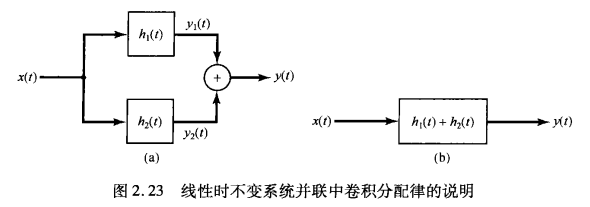# 线性时不变系统的性质

\begin{align*}\newcommand{\dif}{\mathop{}\!\mathrm{d}}\end{align*}

# 冲激响应与LTI系统的性质• 记忆性（是否只与当前时刻有关）：无记忆系统的冲激响应满足：$h[n]=K\delta[n]$，$h(t)=K\delta(t)$
• 可逆性：是否能找到一个逆系统，使得 $h(t)*h_1(t)=\delta(t)$
• 因果性：如果 $h[n]=0,n<0$ 或 $h(t)=0,t<0$，则系统是因果的。因此我们将 $n<0$ 或 $t<0$ 时为零的信号称为 因果信号
• 稳定性：有界信号要产生有界输出，则 $h[n]*x[n]\leq B \sum_{k=-\infty}^{+\infty} \vert h[k] \vert \lt \infty$，$B$是信号的上界，所以稳定系统的脉冲/冲激响应满足：

这个叫绝对可和、绝对可积

# 微分/差分方程描述的LTI系统

1. 求转移算子 $H(p)$
2. 求零输入响应
3. 求零状态响应
1. 求冲激响应
2. 输入与冲激响应相卷积
4. 将零输入和零状态叠加

## 求冲激响应

### 系统方程/算子法

1. 如果 $H(p)$ 是真分式，即 $m\lt n$，并且 $\lambda_1 \neq \lambda_2 \neq \cdots \neq \lambda_n$，则：

我们都可以通个分解成一阶系统之和来求解。

2. 如果 $m\geq n$，则可以利用长除法转化成多项式+真分式
3. 如果存在两个甚至多个 $\lambda$ 相等，可以证明：